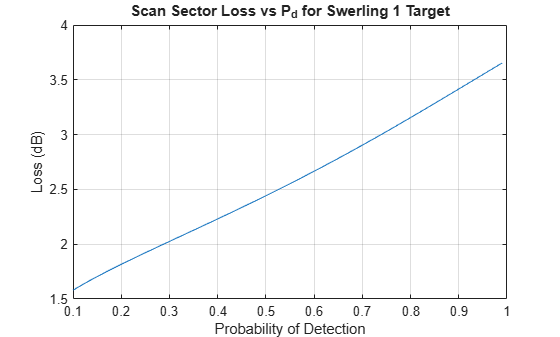# arrayscanloss

Loss due to electronic scanning off broadside

## Syntax

``LSS = arrayscanloss(PD,PFA,N)``
``LSS = arrayscanloss(___,THETAM)``
``LSS = arrayscanloss(___,SW)``
``LSS = arrayscanloss(___,'CosinePower',COSINEPOWER)``

## Description

````LSS = arrayscanloss(PD,PFA,N)` returns the two-way statistical scan sector loss for a radar with a phased array antenna that electronically scans a sector from `-60` to `+60` degrees off broadside. The computation assumes a square-law detector and a nonfluctuating target.```
````LSS = arrayscanloss(___,THETAM)` computes the scan sector loss given the scan sector limits specified about the broadside direction.```

example

````LSS = arrayscanloss(___,SW)` computes the scan sector loss for radar echoes received from a chi-squared distributed target specified by the Swerling case.```
````LSS = arrayscanloss(___,'CosinePower',COSINEPOWER)` specifies the exponent of the cosine modeling the gain loss of an array scanned off broadside. This exponent takes into account two effects that result in the gain reduction due to array scanning. The first effect is the beam broadening due to the reduced projected array area in the beam direction. The second effect is a reduction of the effective aperture area of the individual array elements at off-broadside angles.```

## Examples

collapse all

Compute the statistical scan sector loss for a radar with a phased array antenna. The array scans from `-45` to `70` degrees about the broadside direction. Assume a single pulse is received from a Swerling 1 case target by a square-law detector and the probability of false alarm is set to `1e-6`. Plot the computed loss as a function of the desired probability of detection.

```Pd = 0.1:0.01:0.99; % Detection probabilities Pfa = 1e-6; % Probability of false alarm N = 1; % Number of received pulses ThetaM = [-45 70]; % Scan sector limits Lss = arrayscanloss(Pd,Pfa,N,ThetaM,'Swerling1');```

Plot the statistical scan sector loss.

```plot(Pd,Lss) xlabel('Probability of Detection') ylabel('Loss (dB)') title('Scan Sector Loss vs P_d for Swerling 1 Target') grid on```## Input Arguments

collapse all

Desired probability of detection, specified as a scalar or J-length vector between `0.1` and `0.999999`.

Data Types: `double`

Probability of false alarm, specified as a scalar or K-length vector between `1e-15` and `1e-3`.

Data Types: `double`

Number of received pulses, specified as a positive scalar.

Data Types: `double`

Scan sector limits, specified as a scalar or two-element vector. If `THETAM` is a scalar, then the scan sector spans from `-THETAM` to `+THETAM`. If `THETAM` is a two-element vector of the form ```[theta1 theta2]```, the scan sector spans from `theta1` to `theta2`. The default value is `[-60 60]`. Units are in degrees.

Data Types: `double`

Scan sector limits, specified as the Swerling case for the chi-squared distributed target. The default value of `SW` is `'Swerling0'`.

Exponent of the cosine modeling the gain loss of an array scanned off broadside, specified as a positive scalar. Typically, the exponent value lies between `2` and `3`. The default value is `2.5`.

Data Types: `double`

## Output Arguments

collapse all

Two-way statistical scan sector loss, returned as a J-by-K matrix, where J and K are the dimensions of the `PD` and `PFA` arguments. Units are in decibels (dB).

## Extended Capabilities

### C/C++ Code GenerationGenerate C and C++ code using MATLAB® Coder™.